Balbharti Maharashtra State Board Class 8 Science Solutions Chapter 3 Force and Pressure Notes, Textbook Exercise Important Questions and Answers.

## Maharashtra State Board Class 8 Science Solutions Chapter 3 Force and Pressure

Class 8 Science Chapter 3 Force and Pressure Textbook Questions and Answers

1. Write proper word In the blank space:

Question a.
The SI unit of force is the ………..
(dyne, newton, joule)
The SI unit of force is the newton.

Question b.
The air pressure on our body is equal to the …………. pressure.
(atmospheric, sea bottom, space)
The air pressure on our body is equal to the atmospheric pressure.

Question c.
For a given object, the buoyant force in liquids of different ………… is ………….. .
(the same, density, different, area)
For a given object, the buoyant force in liquids of different density is the same.

Question d.
The SI unit of pressure is ………………
(N/m3, N/m2, kg/m2, Pa/m2)
The SI unit of pressure is N/m2.

2. Make a match.

Question a.

 Group ‘A’ Group ‘B’ 1. Fluid a. Higher pressure 2. Blunt knife b. Atmospheric pressure 3. Sharp needle c. Specific gravity 4. Relative density d. Lower pressure 5. Hectopascal e. Same pressure in all directions

 Group ‘A’ Group ‘B’ 1. Fluid e. Same pressure in all directions 2. Blunt knife d. Lower pressure 3. Sharp needle a. Higher pressure 4. Relative density c. Specific gravity 5. Hectopascal b. Atmospheric pressure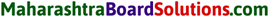3. Answer the following questions in brief.

Question a.
A plastic cube is released in water. Will it sink or come to the surface of water?
It will come to the surface of water.
[Note: This is because its density is less than that of water. When it floats, the unbalanced force acting on it is zero.]

Question b.
Why do the load carrying heavy vehicles have large number of wheels?
The pressure produced by a given force depends on the area of the surface on which the force acts. The greater the surface area, the less is the pressure produced. Load carrying heavy vehicles have large number of wheels so that the load (weight, force) is distributed over large surface area of the wheels in contact with the road. Hence, the pressure decreases and the tyres do not burst.

Try this :
Pressure of a liquid:

Activity 1:
Take a plastic bottle. Take a 10 cm long piece of a glass tube on which a rubber balloon can be fitted. Warm up one end of the glass tube and gently push it into the bottle at about balloon inflates. What is observed? The pressure of water acts on the side of the bottle as well.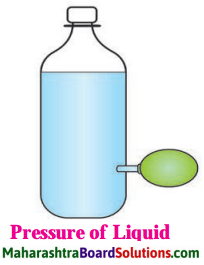[Note: Here, the area of cross section of the tube remains the same. As the level of the water in the bottle rises, the mass of the water increases resulting in increase in the weight. As the applied force increases, the pressure increases. Therefore, the balloon increases in size.]Activity 2:
Take a plastic bottle. Pierce it with a thick needle (or with a hot nail) at the points 1, 2, 3 as shown in the Fig. Fill water in the bottle up to full height. A shown in the figure, water jets will be seen emerging and projecting out. The water jet emerging from the hole at the top will fall closest to the bottle. The jet from the lowest hole falls farthest from the bottle.

Also, jets coming out from the two holes at the same level fall at the same distance from the bottle. What is understood from this? At any one level, the liquid pressure is the same. Also, the pressure increases as the depth of the liquid increases.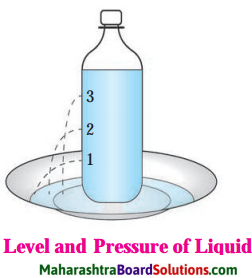Question c.
How much pressure do we carry on our heads? Why don’t we feel it?
The air pressure at the sea level is about 101 × 103 Pa. This is the pressure that we carry on our heads. The cavities in our body are filled with air, and arteries and veins are filled with blood. Their pressure balances the pressure due to the atmosphere. Hence, we don’t feel the atmospheric pressure.

4. Why does it happen?

Question a.
Why does it happen? A ship dips to a larger depth in freshwater as compared to marine water.
The buoyant force acting on a body is proportional to the density of the fluid in which the body is immersed. The density of freshwater is less than that of marine water. Hence, the buoyant force on a body in freshwater is less than that in marine water. Therefore, a ship dips to a larger depth in freshwater as compared to marine water.

Question b.
Why does it happen? Fruits can easily he cut with a sharp knife.

1. It is easy to cut vegetables. fruits with a sharp knife. A blunt knife does not work here.
2. The force exerted perpendicularly on a unit area is called ‘pressure’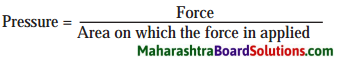3. Presently we are considering only the force acting on an area in a direction perpendicular to it.

Question c.
Why does it happen? The wall of a dam is broad at its base.
1. The pressure at a point in a liquid is proportional to the height of the liquid column above it. Hence, the pressure of water in a dam is much greater at the bottom of the dam than at the top.
2. To withstand this high pressure, the wall of a dam is made stronger and thicker (broad) at the base than at the top.

Question d.
Why does it happen? If a stationary bus suddenly speeds up, passengers are thrown in the backward direction.
1. When passengers sit or stand in a stationary bus, they are in a state of rest. When the bus suddenly speeds up, the lower (parts of their body in contact with the bus acquire the speed of the bus.
2. The upper parts of their body, however, continue to be in the state of rest due to inertia. Hence, they are thrown in the backward direction.5. Complete the following tables.

Question a.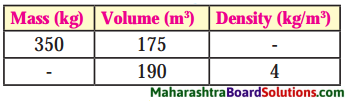Using the formula,
density = mass/volume:

 Mass (Kg) Volume (m3) Density (Kg/m3) 350 175 2 760 190 4

Question b.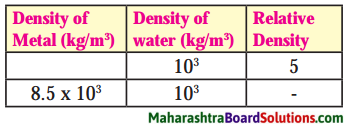Using the formula, relative density = density of a metal/density of water:

 Density of Metal (Kg/m3) Density of water (Kg/m3) Relative Density 5 × 103 103 5 8.5 × 103 103 4

Question c.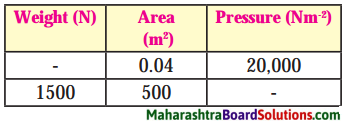Using the formula, pressure = weight/area:

 Weight (N) Area (m2) Pressure (N.m-2) 800 0.04 20000 1500 500 36. The density of a metal is 10.8 × 103 kg/m3. Find the relative density of the metal.

Question a.
The density of a metal is 10.8 × 103 kg/m3. Find the relative density of the metal.
Solution:
Data: Density of the metal = 10.8 × 103 kg/m3
density of water = 103 kg/m3
relative density of the metal = ?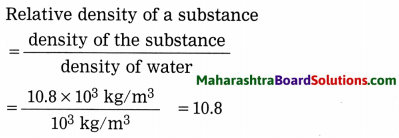The relative density of the metal = 10.8.

7. The volume of an object is 20 cm3 and the mass is 50 g. The density of water is 1 gm-3. Will the object float on water or sink in water?

Question a.
The volume of an object is 20 cm3 and the mass is 50 g. The density of water is 1 gm-3. Will the object float on water or sink in water?
Solution :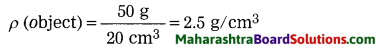It is greater than the density of water.
Hence, the object will sink in water.

8. The volume of a plastic-covered sealed box is 350 cm3 and the box has a mass 500 g. Will the box float on water or sink in water? What will be the mass of water displaced by the box?

Question a.
The volume of a plastic-covered sealed box is 350 cm3 and the box has a mass 500 g. Will the box float on water or sink in water? What will be the mass of water displaced by the box?
Solution: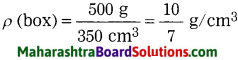It is greater than that of water.
Hence, the box will sink in water.
The volume of water displaced by the box (V) = the volume of the box = 350 cm3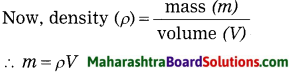∴ The mass of water displaced by the box = 1 g.cm-3 × 350 cm3 = 350 g.

Project:

Question a.
Video record all the experiments (Try it) in this chapter with the help of mobile phone and send to others.

Class 8 Science Chapter 3 Force and Pressure Additional Important Questions and Answers

1. Rewrite the sentences after filling the blanks

Question 1.
The tendency of an object to remain in its existing state is called its …………. .
The tendency of an object to remain in its existing state is called its inertia.

Question 2.
Pressure = ……………Question 3.
1 bar = ……………….. N/m2.
1 bar = 105 N/m2.

Question 4.
1 atmosphere = ………….. Pa.
1 atmosphere = 101 × 103 Pa.Question 5.
The SI unit of density is …………. .
The SI unit of density is kg/m3

Write proper word In the blank space:

Question 1.
According to Archimedes’ principle, the magnitude of the force of buoyancy acting on a body is …………. .
(Vρg, Vρ/g, Vρm, mρg)
According to Archimedes’ principle, the magnitude of the force of buoyancy acting on a body is Vρg.

Question 2.
The pascal is the unit of ……………. .
(velocity, pressure, mass, force)
The pascal is the unit of pressure.

Question 3.
Keeping the surface area constant, if the applied force is doubled, the pressure …………….. .
(becomes double, remains the same, becomes four times, becomes half)
Keeping the surface area constant, if the applied force is doubled, the pressure becomes double.

State whether the following statements are True or False:

Question 1.
The density of water is 1000 g/cm3.
False. [The density of water is 1000 kg/m3 (or 1 g/cm3)]

Question 2.
Force and weight have the same units.
True.

Question 3.
Atmospheric pressure at sea level is about 106 dynes/cm2.
True.Question 4.
The buoyant force due to a liquid is proportional to the acceleration due to gravity.
True.

Question 5.
Atmospheric pressure increases with altitude.
False. (Atmospheric pressure decreases with altitude.)

Question 6.
Pressure due to a given force is directly proportional to the area on which the force acts.
False. (Pressure due to a given force is inversely proportional to the area on which the force acts.)

Question 7.
When a body is completely immersed in a liquid, the buoyant force acting on it due to the liquid is proportional to the volume of the liquid displaced by the body.
True.

Question 8.
The density of a material is useful to determine its purity.
True.

Question 9.
One tends to slip over a banana peel on the street and one can slip due to mud are events that occur due to reduced friction.
True.

Question 10.
Frictional force is electromagnetic in origin.
True.

Identify the odd term:

Question 1.
Density, Pressure exerted by a gas, Mass, Force.
Force. (Force is a vector quantity; other quantities are scalar quantities.)Question 2.
Lactometer, Hydrometer, Voltmeter, Submarine.
Voltmeter. (Its working is not based on Archimedes’ principle. The working of a lactometer, hydrometer and submarine is based on Archimedes’ principle.)

Rewrite the following table in such a way that Column 2 and Column 3 will match with Column 1:

Question 1.

 Column 1 Column 2 Column 3 1. Pressure Mass/volume Specific gravity 2. Density Force/area Decreases with increase in height above the sea level 3. Atmos­pheric pressure No unit Useful to determine the purity of a substance 4. Relative density The pascal Decreases with increase in area

 Column 1 Column 2 Column 3 1. Pressure Force/area Decreases with increase in area 2. Density Mass/volume Useful to determine the purity of a substance 3. Atmos­pheric pressure The pascal Decreases with increase in height above the sea level 4. Relative density No unit Specific gravity

Answer the following questions in one sentence:

Question 1.
Which of the following has more inertia? A ₹ 10 coin and a ₹ 1 coin.
A ₹ 10 coin has more inertia than a ₹ 1 coin.Question 2.
Name the physical quantity expressed in pascal.
Pressure is expressed in pascal.

Question 3.
State the SI unit of pressure.
The SI unit of pressure is N/m2, also called the pascal.

Question 4.
Name the property of a liquid due to which it exerts an upward force on an object immersed in it.
Buoyancy is the property of a liquid due to which it exerts an upward force on an object immersed in it.

Question 5.
Name the principle used in designing ships and submarines.
Archimedes’ principle is used in designing ships and submarines.

Question 6.
What is specific gravity?
The specific gravity of a substance is another name used for relative density, i. e., the ratio of the density of the substance to the density of water.

Question 7.
State any one factor on which the pressure exerted by a liquid at a point inside the liquid depends.
The pressure exerted by a liquid at a point inside the liquid depends on the density of the liquid.

Question 8.
State any one factor on which the buoyant force due to a liquid depends.
The buoyant force due to a liquid depends on the density of the liquid.Question 9.
Name the device used to determine the purity of a sample of milk.
The lactometer is used to determine the purity of a sample of milk.

Question 10.
Name the device used to determine the density of a liquid.
The hydrometer is used to determine the density of a liquid.

Question 11.
Name two instruments whose working is based on Archimedes’ principle.
Working of the lactometer and hydrometer is based on Archimedes’ principle.

Question 1.
Give three examples to show that a force acts on two bodies through an interaction between them.

1. Consider a car at rest on a level (plane) road. If it is pushed from behind, it moves in the forward direction.
2. Iron nails get attracted to the poles of a magnet and stick to the magnet.
3. The moon revolves around the earth.

Question 2.
What is a contact force? Give one example.
A force that acts through a direct contact of two objects or via one more object, is called a contact force.
Example: If a ball at rest on the ground is kicked, it starts moving.Question 3.
What is a non contact force? Give one example.
A force that acts between two objects even if the two objects are not in contact, is called a non contact force.
Example: The earth revolves around the Sun.

Make a list of some more examples in which contact and non contact forces are applied. Write the types of force.
1. Some examples in which contact forces are applied:

• to cut an apple with a knife (muscular force, frictional force)
• to lift a ball lying on the ground (muscular force, frictional force)

2. Some examples in which non contact forces are applied:

• the motion of the earth around the Sun (gravitational force)
• the motion of an electron around the nucleus of an atom (mainly the electric force).

Question 4.
In the following examples, state whether the force is a contact force or non contact force:

1. a reluctant dog is being pulled by his master
2. a boy playing football is kicking the ball away
3. when iron nails are brought near a magnet, they are attracted to the poles of the magnet and stick to the magnet
4. a coconut is falling from the coconut tree
5. when a comb is rubbed against hair, small pieces of paper kept on a table get attracted to the comb
6. when brakes are applied to a moving bicycle, it stops after some time

1. contact force
2. contact force
3. non contact force
4. non contact force
5. non contact force
6. contact force.Question 5.
Give one example in which frictional force is useful.
While walking, we push the ground behind with our feet. In the absence of friction between the ground and the lower surface of our feet, we will slip and will not be able to walk.
[Note: Frictional force is electromagnetic in origin.]

Try this:
Take two plastic bottles with rectangular shape. Close their openings by fitting the lids tightly. Keep two small bar magnets on them and fix them neatly using a sticking tape.

Fill a big plastic tray with water and leave the two bottles floating with magnets at the top. Take one bottle near the other. If the north pole of the magnet is near the south pole of the other magnet, the bottles will head towards each other, because unlike poles attract each other.

Observe what will happen when the directions of the bottles are changed. We can observe change in the motion of the bottles without any direct contact. This means that there exists a non contact force between the two magnets.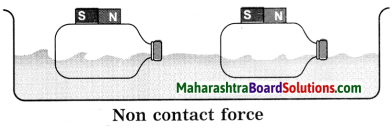Observation: When the directions of the bottles are changed, if the north pole of one magnet is near the north pole of the other magnet (or the south pole of one magnet is near the south pole of the other magnet), the bottles will move away from each other because like poles repel each other.

Question.
You have learnt about static electricity in the previous standard. Electrostatic force is a non contact force. To verify this, which experiment will you perform?Do not switch on the fan in the room. Keep very small pieces of paper on the table. Rub a plastic comb against hair and bring it near the bits of paper. You will find that the bits of paper are attracted by the comb. The comb, on rubbing acquires electrostatic charge. It induces opposite charges on the bits of paper. Hence, the bits of paper are attracted by the comb.

Try this:
Balanced forces and unbalanced force: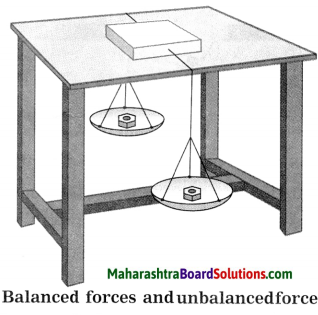Take a cardboard box, tie thick string to its two sides and keep it on a smooth table as shown in Fig. Take the strings on both sides of the table. Tie weighing pans to the two ends. Keep equal masses in both the pans. The box does not move on the table.

If more mass is kept in one of the pans than in the other, the box starts moving in the direction of that pan. Equal gravitational force acts on both the pans when equal masses are kept in them. This means balanced forces act on the box, with effective force equal to zero as these are acting in opposite directions.

On the contrary, if more mass is kept in one pan than in the other, the box starts moving in the direction of the pan with more mass. When unequal forces are applied to the box on the two sides, an unbalanced force acts on the box resulting in imparting motion to the box.

Children playing tug of war pull the rope in their respective directions. If the pull of the force is equal on the two sides, the rope does not move. If the force is more on one side, the rope moves in that direction. This means that initially, the two forces are balanced; the rope moves in the direction of higher force when the forces become unbalanced.

Let us see one more example. When a big grain storage container is required to slide on the ground, it becomes easier if two persons push it rather than one person. When the force is applied by both in the same direction, the movement is easy. You may have experienced this. What do we understand from this example?

1. If several forces are applied on an object in the same direction, a force equal to their addition acts on that object.
2. If two forces are applied on one object in directions opposite to each other, a force equal to their difference acts on the object.
3. A force is expressed in magnitude and direction.

Force is a vector quantity. If more than one force are acting on a body, then the effect on the body is due to the net force. When a force is applied on a stationary object it moves, its speed and direction change. Similarly, a force is required to stop an object in motion.

An object can change its shape due to force. While kneading a dough made from flour, the dough changes its shape when a force is applied. A potter applies a force in a specific direction while shaping the pot. Rubber, when stretched, expands. There are many such examples. ,Question 6.
What are balanced forces?
If a body is acted upon by two forces, equal in magnitude, opposite in direction and having the same line of action, the forces are called balanced forces. Here, the net force acting on the body is zero.

Question 7.
What is an unbalanced force?
If two or more forces act on a body such that their resultant is not zero, the resultant is an unbalanced force.
[Note: Unbalanced force acting on a body = mass of the body × acceleration of the body.]

Question 8.
Explain: Force has magnitude as well as direction. OR Force is a vector quantity.
The effect of force applied to a body depends upon how much force we supply, i.e., the magnitude of the force, and the direction in which the force is applied. Consider a ball at rest on the ground. When ; we push it, it starts rolling. The greater the applied force, the greater is the speed acquired by the ball.

Consider a body moving in a straight line, If we apply a force in the direction of motion of the body, the speed of the body increases. On the contrary, if we apply a force in the direction opposite to that of motion of the body, the speed of the body decreases. These ( examples show that force has magnitude as well as direction, i.e., force is a vector quantity.

Question 9.
Explain the term balanced forces.
Consider a rigid body acted upon by two forces, equal in magnitude, opposite in . direction and having the same line of action. These forces are called balanced forces as their net effect on the body is zero.
Example: A glass slab kept on a table is acted upon by two balanced forces: (i) the weight of the slab acting downward and (ii) the upward force on the slab due to the table. Their net effect on the slab being zero, the slab remains at rest.Question 10.
Explain the term unbalanced force.
A single force acting on a body is an unbalanced force. It produces acceleration in the body. If two or more forces act on a body such that their resultant is not zero, the resultant is an unbalanced force responsible for accelerating the body.
Example: When a ball lying on the ground is hit with a bat, the ball is set in motion by the applied force.

Question 11.
What will happen if the force is removed completely when an object acquires a certain speed?
If the force is removed completely when an object acquires a certain speed, the object will move with the velocity it has at the instant the force is removed.

For example, a body moving with constant speed along a circular path in a horizontal plane will fly tangentially in the sense of motion if the centripetal force (the force directed towards the centre of the circle) is removed completely.

Always remember:

1. The tendency of an object to remain in its existing state is called its inertia.
2. This is why an object in stationary state remains in the same state and an object in motion remain in the state of motion in the absence of an external force.

Types of inertia:

1. Inertia of the state of rest: An object in the state of rest cannot change its state of rest due to its inherent property. This property is called the inertia of the state of rest.

2. Inertia of motion: The inherent property of an object due to which its state of motion cannot change, is called its inertia of motion. For example, a revolving’ electric fan continues to revolve even after it is switched off, passengers sitting in the running bus get aerk in the forward direction if the bus suddenly stops.

3. Directional inertia: The inherent property of an object due to which the object cannot change the direction of its motion, is called directional inertia. For example, if a vehicle in motion along a straight line suddenly turns, the passengers sitting in it are thrown opposite to the direction of turning.

Try this:
Activity 1:
Take a postcard and keep it on a glass. Keep a 5 Rupee coin on it. Now skilfully push the card. The coin straight away falls in the glass. Have you ever done this?
Yes. (Explanation: The postcard moves forward due to the applied force and then falls due to the earth’s gravitational force. In the absence of adequate frictional force between the coin and the postcard, the coin does not move forward with the postcard, but straightaway falls in the glass due to the earth’s gravitational force.)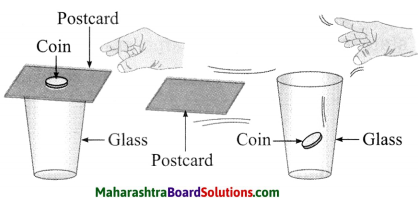Activity 2: Hang a half a kg mass from a stand, with a string 1. Tie another string 2 to the mass and keep it hanging. Now pull the string 2 with a jerk. The string 2 breaks but the mass does not fall. Heavy mass does not move. Now pull the string 2 slowly. The string 1 breaks and the mass fall down. This is because of the tension developed in the string 2 due to the mass.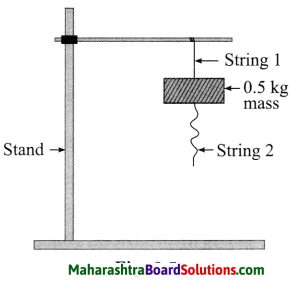(Explanation: (1) As no force acts on the mass, it remains at rest due to inertia. (2) The transmission of force results in the tension in the string 1. As the string 1 cannot withstand it, it breaks and the mass falls down.)Question 12.
What are the three types of inertia?
Types of inertia:

• inertia of rest
• inertia of motion
• inertia of direction.

Question 13.
What is inertia of rest ? Give two examples of inertia of rest.
The inherent property of a body by virtue of which it cannot change its state of rest is called the inertia of rest.
Examples:

1. When we dust a carpet, the carpet moves but the dust particles in it remain at rest due to inertia and hence get separated from the carpet. Hence, the carpet becomes clean.
2. When a bus starts suddenly, the passengers experience a backwarderk due to inertia.

Question 14.
What is inertia of motion? Give two examples of inertia of motion.
The inherent property of a body by virtue of which it cannot change its state of motion is called the inertia of motion.
Examples:

1. When a fan is switched off, its blades continue to rotate for some time. Due to internal friction and friction with air, the blades of the fan stop rotating after some time.
2. Passengers in a bus experience a forwarderk when the bus stops suddenly due to application of brakes.

Question 15.
What is inertia of direction? Give two examples of inertia of direction.
The inherent property of a body by virtue of which it cannot change its direction of motion is called the inertia of direction.
Examples:

1. While sharpening a knife, sparks fly off tangentially in the sense of motion from the grinding stone.
2. When a vehicle moves, the mud particles sticking to its wheels fly off tangentially in the sense of motion. Hence, mudguards are fitted to vehicles.Question 16.
Why do we fall sideways when we are sitting in a bus and it takes a sharp turn?
When we sit in a bus and the bus is in motion, we are in a state of motion in the same direction. When the bus takes a sharp turn, our body tends to maintain the state of motion in the straight line due to inertia. The portion of our body in firm contact with the seat acquires the motion along the curved path, but the upper portion of our body, tends to move in the initial direction of motion. Hence, we fall sideways.

Question 17.
What happens when you shake a wet piece of cloth? Explain your observation.

1. When we shake a wet piece of cloth, water droplets come out.
2. Initially, the wet piece of cloth is at rest. When the cloth is shaken, it is accelerated, but the water droplets in it, due to inertia, tend to maintain the state of rest. Hence, the droplets come out.

Question 18.
If brakes are suddenly applied to a moving car, the passengers in the car are pushed in the forward direction. Explain why.
1. The passengers in a moving car have the same velocity as that of the car. When brakes are suddenly applied to the car, it stops suddenly and the lower parts of the passengers’ bodies in contact with the seats, come to rest.

2. The upper parts of their bodies, however, continue to be in a state of motion due to inertia. Hence, the passengers are pushed in the forward direction.

Question 19.
A person alighting from a moving train is likely to fall in the direction of motion of the train. Explain why.

1. A person in a moving train has the same velocity as that of the train. After alighting from the moving train his feet come to rest on the platform.
2. However, due to inertia, the upper part of his body continues to be in a state of motion in the direction of motion of the train. Hence, he is likely to fall in the direction of motion of the train.

Try this:

Activity 3: Take some sharp pointed nails and push them into a wooden plank by hammering on their heads. Now take? a nail and hold it with its head on the plank and hammer it down from the pointed end. When pressing the drawing pins into a drawing board, they get into the board easily. By applying a force using the thumb one can push the pins into the boards. On the contrary, while pressing ordinary pins into the board with a thumb, the thumb may get hurt.

What does this simple experiment tell?
The nail easily penetrates into wood from its pointed end. From this you will notice that when a force is applied on the head of the nail, it is easy to hammer it into the plank.
Explanation: The less the area of the surface on which the force is applied, the greater is the effect of the force.Question 20.
Define pressure.
The force exerted perpendicularly on a unit area is called pressure.

Question 1.
It is easy to cut vegetables, fruits with a sharp knife. A blunt knife does not work here. Why does this happen?
The effect of a given force varies l inversely as the area of the surface on ( which the force is applied. The less the surface area, the greater is the effect of the force. The cutting edge of a sharp ’ knife has less cross sectional area relative to that of a blunt knife. Hence, it is easy to cut vegetables, fruits with a sharp knife, rather than with a blunt knife. For a given force, pressure is inversely proportional to the area of the surface on which the force acts.

Question 21.
State the formula for pressure, Hence, determine the unit of pressure.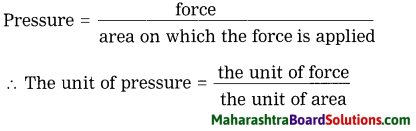The SI unit of force is the newton (N) and that of area is m2. Therefore, the SI unit of pressure is N/m2. It is called the pascal (Pa). 1 Pa = 1 N/m2.
[Note: The unit pascal is named in honour of Blaise Pascal (1623-62), French mathematician, physicist and philosopher.]

Question 22.
State the CGS unit of pressure. State the relation betweeen the SI and CGS units of pressure.
The CGS unit of pressure is the dyne/cm2.
IN = 105 dynes, 1m2 = (102 cm)2 = 104 cm2 1 Pa = 1 N/m2 = 105 dynes/104 cm2 = 10 dynes/cm2.

Question 23.
State the factors on which pressure depends.
Pressure depends on the applied force and the area of the surface on which the force is applied.Question 24.
Explain with a suitable example that pressure varies inversely as the area of the surface on which the force is applied, if the force remains constant.
The tip of a pointed nail has an extremely small area, while that of a blunt nail has a comparatively large area. A given force creates a large pressure on the pointed nail and it can be easily hammered into the wood, while a very less pressure is created on the blunt nail and it cannot be easily hammered into the wood.

This shows that pressure varies inversely as the area of the surface on which the force is applied, if the force remains constant. If the same force is applied to surfaces having different areas, the pressure is more on the surface having a smaller area.

[Note: The bottom surface of a camel’s feet is broad. Hence, the camel’s weight acts on a large surface area. This reduces the pressure on the sand. Hence, the camel’s feet do not penetrate deep into the sand. Therefore, it becomes easier for the camel to walk on sand.]

Question 25.
With neat diagrams, describe an experiment to show that pressure increases if the surface area is decreased, keeping the applied force the same.
Take a brick measuring 20 cm x 10 cm x 5 cm. Take some clay in a glass trough. Add water to it and knead it into a soft dough. Place the brick on the dough with one of its faces measuring 20 cm x 10 cm in contact with the dough. Observe how deep the brick penetrates into the dough.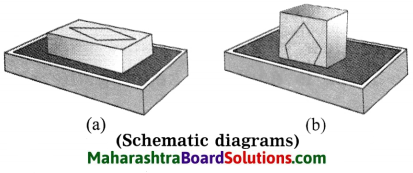Clean the brick and place it on the dough with one of its faces measuring 10 cm x 5 cm in contact with the dough. Observe how deep the brick penetrates into the dough. You will find that the brick penetrates deeper in this case than that in the first case.

• In the first case, the weight of the brick acts on a surface area of 200 cm2.
• In the second case, the weight of the brick acts on a surface area of 50 cm2.
• This shows that pressure increases if the surface area is decreased, keeping the applied force the same.Question 26.
State the unit for pressure used in atmospheric science. How is it related to the unit pascal?
In atmospheric science, the unit used for pressure is the bar. 1 bar = 10 Pa (pascal).

Try this:

Question 1.
Do the activity as depicted in Figure What is seen?
In Fig.(a), the plank is horizontal. In Fig.(b), four books are placed side by side on the plank. The plank bends slightly due to the pressure produced by the weight of the books.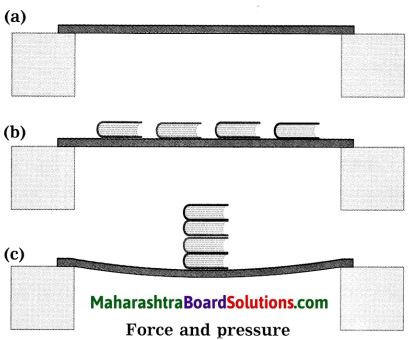In Fig.(c), the four books are placed one above the other in the middle of the plank. Here the area of the surface of the plank on which the force acts is reduced by a factor of four relative to the earlier case. Hence, the plank bends considerably.
[Note: This shows that for a given force, pressure varies inversely as the area of the surface on which the force acts.]

Question 1.
You must have seen a vegetable vendor carrying a basket on her head. She keeps a twisted piece of cloth on the head, below the basket. How does it help?
Keeping a twisted piece of cloth on the head increases the area of the surface on which the weight of the basket containing vegetables acts. Hence, the pressure produced by the force (weight) is reduced and it becomes easier to carry the basket.

Question 2.
We cannot stand at one place for a long time. How then can we sleep on a place for 8 and odd hours?
When we stand, our weight acts on relatively small surface area, resulting in increased tension on the muscles of our legs. Hence, we cannot stand at one place for a long time. When we sleep, our weight acts on relatively large surface area, resulting in comparatively reduced tension. Therefore, we can sleep on a place for 8 and odd hours.

Question 3.
For skiing on ice, why are long flat skis used?
The pressure produced by a given force depends on the area of the surface on which the force acts. The greater the surface area, the less is the pressure produced. The skis used to slide over snow are long and flat so that the area is increased and hence the pressure is decreased. This makes it easier to slide over snow.Question 27.
Why do the blades of a pair of scissors have sharp edges?
The pressure produced by a given force depends on the area of the surface on which the force acts. The less the surface! area, the greater is the pressure produced, The blades of a pair of scissors have sharp edges so that the area is decreased and hence the pressure is increased. This makes cutting • an object such as cloth easier.
[Note: Answers to questions such as why is the blade of an axe sharp? or why is the blade of a saw sharp? can be written on the basis of the answer given above.]

Question 28.
Why does a needle have s sharp point?
The pressure produced by a given force depends on the areas of the surface on which the force acts. The less the surface area, the greater is the pressure produced. A needle has a sharp point so that the area is decreased and hence the pressure is increased. This makes the action of piercing easier.

Question 29.
Why do school bags have broad shoulder straps?
The pressure produced by a given force depends on the area of the surface on which the force acts. The greater the surface area, the less is the pressure produced. School bags have broad shoulder straps so that the weight of the bag is distributed over a large surface area thereby decreasing the pressure on the shoulders of the student carrying the bag.

Question 30.
How will the pressure change if the area is doubled keeping the force the same?
If the area is doubled, keeping the force the same, the pressure will become half the initial pressure.

Question 31.
How will the pressure change if the force is doubled, keeping the area the same?
If the force is doubled, keeping the area the same, the pressure will become double the initial pressure.

Question 32.
State the characteristics of the pressure due to a liquid (or a fluid in general).
OR
Write a short note on the pressure due to a liquid (a fluid in general).
Characteristics of the pressure due to a liquid (or a fluid):

1. The pressure at a point in a liquid (or a fluid) is due to the weight of the liquid (fluid) column above that point.
2. It acts on all sides of the container.
3. At a given depth it is the same in all directions.
4. It is independent of the size and shape of the container.
5. It is proportional to the height of the liquid (fluid) column above the given point.
6. It is proportional to the density of the liquid (fluid).
7. It is proportional to the acceleration due to gravity at the given place.

[Note: The pressure exerted by a liquid (or gas or fluid) at a depth h below the free surface of the liquid = hpg, where p is the density of the liquid (or gas or fluid) and g is the acceleration due to gravity.]Question 33.
Give two examples to show that air exerts equal pressure in all directions.

1. When air is filled in a balloon, it acquires its characteristic shape such as round or oval.
2. When a bicycle tube is filled with air, it acquires its characteristic (tube-like) shape throughout. This shows that air exerts equal pressure in all directions.

Question 34.
Whatisafluid?Givetwoeamples.
A fluid is a substance which can flow.
Examples:

1. Water (liquid)
2. Air (gas)

[Note: Liquids and gases together are called fluids. Gases have very low viscosity compared to liquids. A liquid with low viscosity flows easily. A liquid with high viscosity does not flow easily.]

Question 35.
Take two rubber balloons. Fill one with water and blow air into the other. Now prick both balloons with a pin. What do you observe?
Water and air both come out of the balloons. Air escapes quickly compared to water and produces a loud sound.

Question 36.
State the characteristics of pressure exerted by a fluid.
OR
Write a short note on pressure exerted by a fluid.
Characteristics of pressure exerted by a fluid:

1. A fluid due to its weight, exerts pressure on the base as well as the walls of the container that holds it.
2. A fluid exerts pressure on a body immersed in it.
3. The pressure exerted on any confined mass of fluid is transmitted undiminished in all directions.

[Note: The pressure exerted by a fluid is a scalar quantity.]Question 37.
Take an empty can. Pour small quantity of water in it. Boil this water for a few minutes until the steam has driven out most of the air. Now close the can with the stopper tightly. Allow it to cool by pouring cold water over it. What do you observe?
[Note: The steam inside the can condenses to form water as the can cools. Therefore, the pressure inside the can becomes much less than the external pressure of the air. Hence, the can gets crushed.]

Question 38.
Put a folded newspaper on a plastic bag. Blow air into the bag. What do you observe?
The plastic bag inflates as air is blown into it. This raises the folded news¬paper put on the bag.

Question 39.
What is meant by atmospheric pressure?
The earth is surrounded by air from all sides. This layer of air is called the atmosphere. Its density is high up to about 16 km from the earth’s surface. Beyond that, up to about 400 km, its density is very low. Air, due to its weight, exerts pressure on the surface of the earth.

The pressure exerted by air or the atmosphere surrounding the earth is known as the atmospheric pressure. It is the ratio of the weight of the air to the area of the surface of the earth. It decreases with altitude as the density of air decreases with altitude and also the weight of the air column above a given place.
[Note: At sea level the atmospheric pressure is about 105 Pa. We do not feel it because the pressure of blood and other fluids in our body balances it.]Question 40.
State the relation between 1 atmosphere and the pascal.
1 atmosphere = (about) 101 × 103 Pa (pascal)
[Note: The air pressure at the sea level is (about) 1 atmosphere.
1 atmosphere = 101325 Pa.
1 bar = 103 mbar (millibar)
1 mbar w 103 Pa (hectopascal)
Atmospheric pressure is expressed in mbar or hectopascal (hPa).]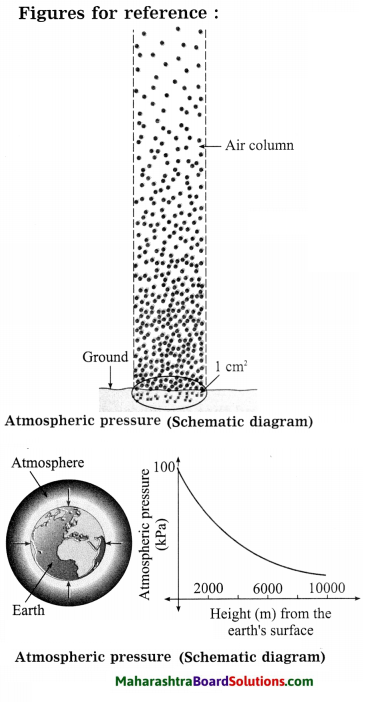Question 1.
At the sea level the atmospheric pressure 101 × 103 Pa is acting on a table top of size 1 m2. Under such a heavy pressure, why doesn’t the table top crumble down?
The air below the table top exerts pressure 101 × 103 Pa on it in the upward direction. Hence, the table top doesn’t crumble down.

Question 41.
Think – Why?
Question i.
Some people feel their ears popping at the top of a mountain.
Atmospheric pressure decreases with altitude. At the top of a mountain, it becomes less than the internal pressure in the ear. Hence, some people feel their ears popping at the top of a mountain.

Question ii.
Some people feel breathless as they climb higher and higher on a mountain.
Atmospheric pressure decreases with altitude. Hence, some people feel breathless as they climb higher and higher on a mountain.

Question iii.
We can enjoy a cold-drink or fruit juice with the help of a straw but can we imagine drinking a cold-drink or fruit juice on the moon using a straw?
When we suck air in the straw, the pressure of the air in the straw becomes less than that of the outside air on the cold drink or fruit juice in the bottle (or the glass). Hence, the cold drink or fruit juice rises in the straw and enters our mouth. We can then drink it. There is no atmosphere on the moon. Hence, it is not possible to enjoy a cold drink or fruituice on the moon by using a straw.

Question iv.
People are often advised not to carry fountain pens while travelling by air.
The ink in a fountain pen (filled at sea level at atmosphere pressure) may come out through its mouth while travelling by air if the outside pressure becomes less than the pressure in the ink holder of the pen. This can spoil the clothes/purse/bag. Hence, people are often advised not to carry fountain pens while travelling by air.Question 42.
Why do some people have earache when they travel by an aeroplane?
When an aeroplane descends at a high speed, there is an increase in air pressure. This increases the pressure on the ear drum. Hence, some people have earache when they travel by an aeroplane.

Question 43.
Explain why a person may bleed from the nose when at a great height above the sea level.
The pressure exerted by the blood in blood capillaries is slightly more than the atmospheric pressure and acts in a direction opposite to that of the atmospheric pressure. Atmospheric pressure decreases with height and at a great height above the sea level, it is very low.

As a result, there arises a difference in the internal and external pressures on the walls of the cells and blood capillaries. If the difference is large, it may cause the cell wall and the blood capillaries to burst. Thus, the capillaries in the nose (and ear) may burst causing bleeding.

Question 44.
When a rubber sucker is pressed onto a flat glass surface, it sticks tightly on the surface. Why? You need a large force to separate it from the surface. Why?
When a rubber sucker is pressed onto a flat glass surface, practically all the air between the surfaces of the sucker and the glass is pushed out. The air pressure there becomes much less than the atmospheric pressure. Hence, the sucker sticks to the glass due to the external atmospheric pressure. The atmospheric pressure is about 105 Pa. It is very large. Hence, to work against it to separate the sucker from the glass, a large force is needed.

Question 45.
Describe a simple experiment to demonstrate atmospheric pressure.
Fill a glass completely with water (to its brim) and cover it with a flat and stiff card paper (or a piece of glass). Holding your palm on the card, turn the glass upside down and take the palm away from the card.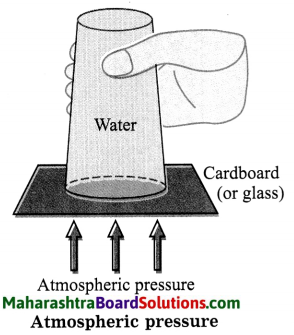You will find that the water does not spill. The atmospheric pressure on the card (acting upward) is greater than the pressure of the water in the glass (acting downward). Hence, the water in the glass does not spill.

Question 46.
Explain the working of an ink dropper.
[Application]
An ink dropper consists of a tube of glass or plastic, with one end tapering to a narrow opening and the other end fitted with a small rubber bulb. When the narrow open end is dipped into the ink and the rubber bulb is pressed, some air in the tube escapes through the open end. This reduces the air pressure inside the dropper.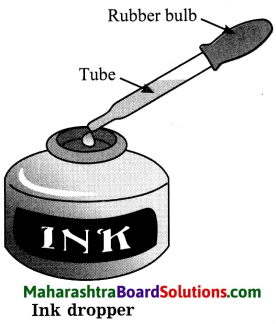On releasing the bulb, the atmospheric pressure on the ink pushes the ink into the dropper. The dropper is then taken out and its open end is held over the open barrel of the pen. The bulb is then pressed so that the ink in the dropper enters the pen.
[Note: The medicine dropper works in the same manner.]Question 47.
Why is the opening of a dropper very narrow?
The pressure produced by a given force is inversely proportional to the area of the surface on which the force acts. The opening of a dropper is very narrow. Hence, its area of cross-section is very small. As a result, even if the dropper has a small amount of ink it, its pressure can equal the atmospheric pressure. As the opening is narrow, it is easier to transfer the ink to the pen. The possibility of ink spilling is very low.

Question 48.
What is the characteristic of the cap of eye drop bottles?
The cap of an eye drop bottle is fitted with a dropper.

Question 49.
Explain the working of a syringe
used by children when they play with coloured water. [Application]
As shown in the figure, a syringe used by children when they play with coloured water consists of a cylinder made of plastic or metal fitted with a piston. One end of the cylinder is in the form of a narrow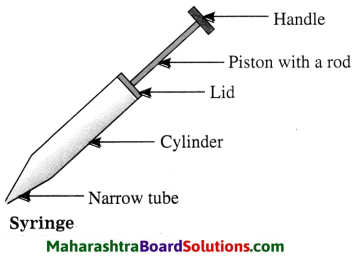tube. The snugly fitting piston can slide in , and out smoothly. The rod connected to the i piston passes through a hole in the centre of the lid and has a handle at the other end. When the tip of the narrow tube is dipped in coloured water (or any other liquid) and the piston is pushed towards the tip, up to the bottom, most of the air in the cylinder escapes through the tube, reducing the pressure.

When the piston is moved up, the coloured water rises in the part of the cylinder below the piston due to the atmospheric pressure. Finally, the inner pressure equals the atmospheric pressure and no more coloured water enters in or comes out.

To spray the coloured water, the tube is taken out and the piston is moved towards the opening of the tube. As the inner pressure is now greater than the atmospheric pressure, the coloured water gushes out of the narrow opening of the tube.Question 50.
How does the doctor’s syringe work?
As shown in the figure, a syringe used by children when they play with coloured water consists of a cylinder made of plastic or metal fitted with a piston. One end of the cylinder is in the form of a narrowtube. The snugly fitting piston can slide in , and out smoothly. The rod connected to the i piston passes through a hole in the centre of the lid and has a handle at the other end.

When the tip of the narrow tube is dipped in coloured water (or any other liquid) and the piston is pushed towards the tip, up to the bottom, most of the air in the cylinder escapes through the tube, reducing the pressure. When the piston is moved up, the coloured water rises in the part of the cylinder below the piston due to the atmospheric pressure.

Finally, the inner pressure equals the atmospheric pressure and no more coloured water enters in or comes out. To spray the coloured water, the tube is taken out and the piston is moved towards the opening of the tube. As the inner pressure is now greater than the atmospheric pressure, the coloured water gushes out of the narrow opening of the tube.

The tip of a syringe is fitted with a very fine and hollow needle. The required quantity of medicine can be taken in the syringe with the help of the piston. The medicine can then be injected into the body of a patient using the needle and the piston.

Question 51.
Explain the origin of pressure due to a gas enclosed in a container.
Molecules of a gas are in a state of continuous random motion. These molecules possess energy due to motion. When the molecules collide with a wall of the container, they rebound. A large number of molecules collide with the wall every second. Hence, a significant force is exerted on the wall. Pressure is the force per unit surface area. Thus, a pressure is exerted by the gas on a wall of the container.

Try this:

Buoyant force:
Take a plastic bottle and fix the lid tightly. Now place it in wafer and see. It will float on water. Try and push it into the water. Even when pushed, it continues to float. This experiment can also be done with a plastic hollow ball. Now fill the bottle with water to the fullest capacity and close the lid, and release in water. The bottle will float inside the water. Why does this happen?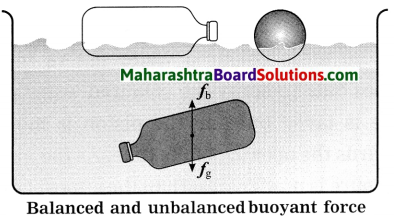The empty plastic bottle floats on the surface of water. On the contrary, the bottle full of water floats inside water but does not go to the bottom. The weight of the empty bottle is negligible as compared with the weight of the water inside.

Such a bottle with water neither floats on the surface, nor does it go to the bottom. This means the force due to gravity acting downwards (fg), must have been balanced by an opposing force in the upward direction (fb) on the bottle filled with water. This force must have originated from the water surrounding the bottle. The upward force acting on the object in water or other fluid or gas is called buoyant force (fg).Question 52.
Define buoyant force.
The upward force acting on the object in water or other fluid or gas is called the buoyant force.

Question 53.
State the factors on which the buoyant force depends.
The buoyant force depends upon the volume of the object immersed in the fluid (V), the density of the fluid (ρ1) and the acceleration due to gravity (g) at that place.
[Note: Magnitude of the buoyant force = Vρ1 g.]

Question.
While pulling a bucket from a well, the bucket full of water immersed fully in water appears to weigh less than when it has been pulled out of water. Why?
1. When a bucket full of water is immersed in water, the net force acting on the bucket = weight of the bucket full of water-the buoyant force exerted by the water on the bucket. The buoyant force due to a fluid is proportional to the density of the fluid.

2. The density of water is much greater than that of air. Therefore, the buoyant force acting on a bucket full of water while it is in water is much greater than that when it is out of water, i.e., in air. Hence, it appears to weigh less, while it is in water than when it has been pulled out of water.

Try this:

Question 1.
Take a piece of thin aluminum sheet and dip it in water in a bucket. What do you observe?
It sinks in the water.

Question 2.
Now shape the same piece of aluminium into a small boat and place it on the surface of water. It floats, isn’t it?
Yes, the boat floats on the surface of water.Question 54.
A lemon sinks in a glass filled with water but it floats when we stir in two spoons of salt in the water. Explain why.
When salt is dissolved in water, the density of the water increases. The buoyant force is proportional to the density of the liquid. Hence, when the lemon is immersed in the water containing salt, the magnitude
of the buoyant force acting on the lemon becomes greater than the magnitude of the weight of the lemon. Therefore, it floats in water.

Do you know?

How is it decided that an object left in a liquid will get sink in the liquid, will float on the surface, or will float inside the liquid?
1. The object floats if the buoyant force is larger than its weight.
2. The object sinks if the buoyant force is smaller than its weight.
3. The object floats inside the liquid if the buoyant force is equal to its weight. Which forces are unbalanced in the above cases?
Unbalanced force:
1. Magnitude of the unbalanced force acting on the object = magnitude of the buoyant force on the object-magnitude of the weight of the object. The direction of the unbalanced force is the direction of the buoyant force.

2. Magnitude of the unbalanced force on the object = magnitude of the weight of the object – magnitude of the buoyant force on the object. The direction of the unbalanced force is the direction of the weight of the object.

3. Here, the unbalanced force is zero.

Try this:

Take a long rubberband and cut it at one point. At one of its ends tie a clean washed stone or a 50 g weight as shown in Figure
Now hold the other end of the rubberband and make a mark there. Keep the stone hanging in air and measure the length of the rubberband from the stone to the mark made earlier.

Now take water in a pot and hold the rubberband at such a height that the stone sinks in it. Again measure the length of the rubberband up to the mark. What is observed? This length is shorter than the earlier length. While dipping the stone in water, length of the stretched rubber gets slowly reduced and is minimum when it sinks completely. What could be the reason for a shorter length of the rubberband in water?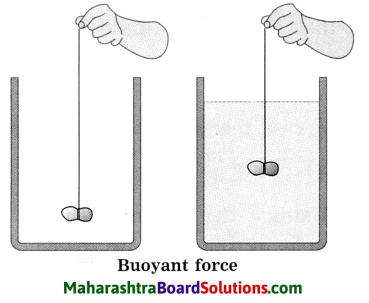When the stone is sunk in water, a buoyant force acts on it in the upward direction. The weight of the stone acts downwards. Therefore, the force which acts on it in the downward direction is effectively reduced.
(This decreases the downard force on the rubberband. Hence, its length decreases.)

Question 55.
State Archimedes’ principle.
Archimedes’ principle: When an object is partially or fully immersed in a fluid, a force of buoyancy acts on it in the upward direction. This force is equal to the weight of the fluid displaced by the object.
[Note: The two forces mentioned here are equal in magnitude and opposite in direction.]Question 56.
Using Archimedes’ principle, determine the magnitude of the buoyant force.
Let m = mass of the body (object) immersed in the fluid, V = volume of the body, ρ = density of the body, ρ1 = density of the fluid, g = magnitude of the acceleration due to gravity. Suppose that the body is completely immersed in the fluid. Then the volume of the fluid displaced by the body = V. According to Archimedes’ principle, magnitude of the buoyant force = magnitude of the weight of the fluid displaced by the body = mass of the displaced fluid × g = volume of the displaced fluid × density of the fluid × g
(as density = mass/volume)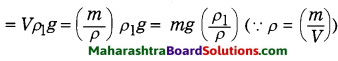If the body is partially immersed in the fluid, the volume of the fluid displaced by the immersed part of the body = xV ; here 0 < x < 1.
In this case, the magnitude of the buoyant force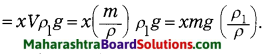Question 57.
Using the formula for the magnitude of the buoyant force, state under what conditions the body will
1. sink in the fluid
2. float in the fluid
3. remain in equilibrium anywhere within the fluid.
Magnitude of the buoyant force on the body = mg $$\left(\frac{\rho_{1}}{\rho}\right)$$
= magnitude of the weight of the body × $$\left(\frac{\rho_{1}}{\rho}\right)$$
1. If the density of the fluid (ρ1) is less than the density of the body (ρ), the magnitude of the buoyant force on the body will be less than the magnitude of the weight of the body. Therefore, the body will sink in the fluid.|

2. If the density of the fluid is greater than that of the body, the magnitude of the buoyant force on the body will be greater than that of the weight of the body. Therefore, the body will float in the fluid.

3. If the density of the fluid is equal to that of the body, the magnitude of the buoyant force on the body will be equal to that of the weight of the body. Therefore, the body will remain in equilibrium anywhere within the fluid.

Question.
Explain the observations in the earlier experiments according to the Archimedes’ principle.
The increase in the length of the rubberband (y) is proportional to the downward force (f) acting on it.
1. When the stone tied to the rubberband is hanging in air, the magnitude of the buoyant force exerted by air on the stone is negligible compared to that of the weight of the stone. Hence, ignoring it, we have f = fg = mg, where m is the mass of the stone and g is the acceleration due to gravity.

2. When the stone is immersed partially in water, f = fg – fb, where fb is the magnitude of the buoyant force exerted by water on the stone.
Now, fg = Vρg and fb = xVρwg, where V = volume of the stone, ρ = density of the stone, xV= volume of the water displaced by the part of the stone immersed in water = volume of the part of the stone immersed in water (x < 1) and ρ = density of water.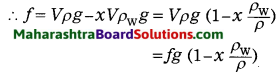This shows that as the stone is gradually lowered in water, x goes on increasing and hence/goes on decreasing. Therefore, elongation (y) of the rubberband goes on decreasing, i.e., the length of the rubberband goes on decreasing.

3. When the stone is completely immersed in water, x becomes maximum, equal to 1. Here, f = fg $$\left(1-\frac{\rho_{\mathrm{W}}}{\rho}\right)$$. This is the minimum value of. Here, the elongation of the rubberband is minimum, i.e., the length of the rubberband is minimum.Question 58.
State the applications of Archimedes’ principle.
Archimedes’ principle is used in the construction of ships and submarines. The working of the lactometer and hydrometer is based on Archimedes’ principle.
[Note: The hydrometer is used to determine the density or relative density of a liquid.]

Question 59.
If a spring balance is used to weigh a body, will the weight of the body be the same in vacuum and air? Explain why.
When a body is suspended in air, the buoyant force acts on the body. Hence, the magnitude of the net downward force on 1 the body = the magnitude of the weight of the body – the magnitude of the buoyant force on the body. Hence, when a spring balance is used to weigh a body, the weight of the body in air is less than that in vacuum.

Question 60.
What is density of a substance? I Obtain its SI unit.
The density of a substance is the ratio of its mass to volume.
The SI unit of density = $$\frac{\text { the SI unit of mass }}{\text { the SI unit of volume }}$$ = kg/m3
[Note: Density is useful in determining the purity of a substance. The CGS unit of density is g/cm3.
1 kg/m3 = 103g/(100 cm)3 = 10-3g/cm3
∴ 1g/cm3 = 1000 kg/m3]

Question 61.
What is relative density of a substance?
The relative density of a substance is the ratio of its density to the density of water.
[Note: Relative density is also called specific gravity. It is a ratio of two equal (same) physical quantities. Hence, it has no unit.]

Question 62.
A piece of wood floats both in water and kerosine. In which liquid does it sink more during floating? Why?
The piece of wood sinks more in kerosine than in water during floating. The density of kerosine is less than that of water. The buoyant force on a body is proportional to the density of the liquid in which the body is immersed. When a body floats, the magnitude of the buoyant force acting on the body is equal to that of the weight of the body.

Hence, the volume of the liquid displaced by a floating body is inversely proportional to the density of the liquid. As a result, when a piece of wood floats in kerosine, it displaces greater volume of kerosine compared to the volume of water displaced when the piece of wood floats in water. Hence, it sinks more in kerosine than in water.
[Note: When a body floats in a liquid fb = fg
∴ Vρ1g = Vbρbg
∴ The volume of the liquid displaced by the body is V = Vb $$\frac{\rho_{\mathrm{W}}}{\rho_{1}}$$, where Vb is the volume of the body, ρb is the density of the material of the body and ρ1 is the density of the liquid. Thus, V ∝ 1 /ρ1]Question 63.
State whether the body will float or sink in a liquid if the density of the body is 1. greater than that of the liquid 2. less than that of the liquid 3. equal to that of the liquid.

1. The body will sink in the liquid if the density of the body is greater than that of the liquid.
2. The body will float in the liquid if the density of the body is less than that of the liquid.
3. The body will float inside the liquid if the density of the body is equal to that of the liquid.

Question 64.
If the relative density of a body is greater than 1, will it float in water?
If the relative density of a body is greater than 1, it will not float in water.
[Note: The relative density of a pin is much greater than 1. But when kept gently on the surface of water, it floats. This is due to the surface tension of water.]

Question 65.
A glass of water has an ice cube floating in water. The water level must touches the rim of the glass. Will the water overflow when the ice melts? Give the reason.
The water will not overflow when the ice melts. The water level will remain the same. Ice floats on water because its density is less than that of water. When ice melts, the volume of the water formed is less than the volume of the ice which has melted.

When ice in water melts, this difference equals the volume of the water formed when the part of ice above the surface of water melts. Therefore, the water level remains the same. Hence, there is no overflow of water when the ice melts.

Question 66.
A plastic ball is released underwater. State whether it will sink or come up to the surface of water. Give the reason.
A plastic ball released under water will come up to the surface of water. The density of water is greater than that of plastic. Hence, when a plastic ball is under water, the magnitude of the buoyant force exerted by water on the ball is greater than the magnitude of the weight of the ball.

Therefore, the ball will start moving upward. As it comes up with part of the ball above the water surface, the volume of the water displaced by the ball becomes less and hence at a certain stage, the buoyant force and the weight balance each other. Then the ball continues to remain in that state, as the net force on the ball becomes zero.
[Note: Initially, the ball moves slightly up and down near the water surface. The force due to friction with water, opposing the motion of the ball, finally makes the ball steady.]

Write short notes:

Question 1.
Buoyant force.
1. When a body is immersed partially or completely in a liquid, the liquid exerts forces on all sides of the body. This force is perpendicular to the surface of the body and equals the product of pressure and area at that point.

2. The resultant of all these forces acts upward. It is called the upthrust or buoyant force.

3. The buoyant force is proportional to (i) the volume of the liquid displaced by the body (ii) the density of the liquid (iii) the acceleration due to gravity. Its magnitude equals the magnitude of the weight of the liquid displaced by the body.Question 2.
Applications of Archimedes’ principle.
1. The working of a lactometer, a device used to determine the purity of a sample of milk, and a hydrometer, a device used to determine the density of a liquid, is based on Archimedes’ principle. The extent to which a lactometer floats (or sinks) depends on the density (and hence purity) of the milk. The same thing is true for a hydrometer. The greater the density of a liquid, the less is the extent to which a body sinks in it.

2. Archimedes’ principle is used in design of ships and submarines. A submarine is provided with large tanks at the front and the back. Its weight can be increased by filling the tanks with sea water or air from compressed air reservoirs. The weight can be decreased by pumping out water from the tanks by forcing compressed air in them. By controlling the weight, it can be made to sink or rise to the surface as desired.

3. The density of a body that floats or sinks in water or kerosine can be determined by. Archimedes’ principle.
4. The density of kerosine can be determined by Archimedes’ principle, using a body of material that is not affected by water and kerosine.

Give scientific reasons:

Question 1.
The tiles are placed over a slushy patch of ground to help cross It.

1. Tiles have greater area than the area of our feet.
2. The weight of the person crossing the slushy patch is exerted over a large area of the tiles.
3. Therefore, there is a decrease in the pressure and hence the tiles do not sink much in the slushy patch of ground. This helps to cross the slushy patch of ground.

[Note: If there were no tiles, the feet will come in direct contact with the slushy ground. The area of the feet being less, the weight of the person will act over a smaller area. Therefore. there will be more pressure and hence the feet will sink into the slushy ground.]

Question 2.

1. The head of a drawing pin is flattened and the other end is pointed.
2. When enough force is applied to the head of the pin, the pressure due to the force on the pointed end increases tremendously and the pin can be easily inserted in the drawing board.
3. When we press the flattened end, the force applied spreads over a larger area. This reduces the pressure of the reaction force acting on the thumb. Hence, the thumb is not injured.
4. If the head of the pin is sharp, then the pressure due to the force would be more and hence the pressure of the reaction force would also be more and the sharp end would prick the thumb causing injury.

Question 3.
An iron nail sinks in water but a ship made from iron floats on water.
1. An iron nail sinks in water because its density is more than that of water.
2. A ship made from iron, due to the particular shape given to it, displaces a large amount of water so that the buoyant force acting on the ship due to water balances the weight of the ship. Hence, the ship floats on water.Question 4.
A piece of iron sinks in water but floats on mercury.
The density of iron is more than that of water but less than that of mercury. Hence, a piece of iron sinks in water but floats on mercury.

Question 5.
A sheet of metal that sinks in water can float if shaped like a pan.

1. A sheet of metal sinks in water because its density is more than that of water.
2. If the sheet is shaped like a pan, it can displace a large amount of water such that the buoyant force on the pan due to water balances the weight of the pan. Hence, it can float on water.

Solve the following examples:

Problem 1.
(i) Calculate the pressure exerted by the wooden block when it is kept in the vertical position.
Given: The length of the wooden block is 80 cm, the breadth is 50 cm, the thickness is 20 cm and the weight is 500 N
(ii) Also calculate the pressure when the wooden block is kept in the horizontal position with its surface 80 cm × 50 cm touching the floor.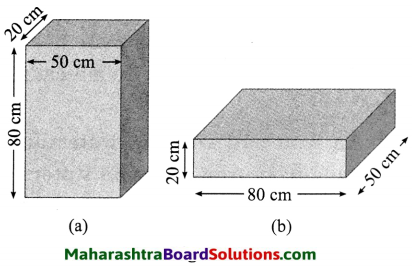Solution:
Data: F = W = 500 N, l = 80 cm = 0.8 m, b = 50 cm = 0.5 m. h = 20 cm = 0.2 m
(i) A = bh = 0.5 m × 0.2 m = 0.1 m2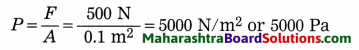The pressure exerted in the vertical position of the block = 5000 N/m2 or 5000 Pa.

(ii) A = lb = 0.8 m × 0.5 m = 0.4 m2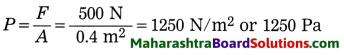The pressure exerted in the horizontal position of the block = 1250 N/m2 or 1250 Pa.

Problem 2.
Measure the length, breadth, height and mass of a rectangular tiffin box. Find the weight of the box and calculate the pressure in two different positions as in Ex. (1) above.
Solution:
Let l = 0.25 m, 6 = 0.1 m, h = 0.05 m, F = W= 0.5 N
(i) A = bh = 0.1 m × 0.05 m = 0.005 m2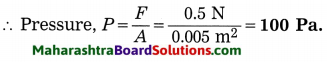(ii) A = lb = 0.25 m × 0.1 m = 0.025 m2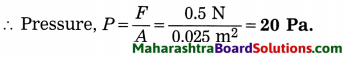Problem 3.
A force of 1000 N is applied over an area 50 cm × 20 cm. Find the corresponding pressure.
Solution:
Data: F = 1000 N,
A = 50 cm × 20 cm = 0.5 m × 0.2 m = 0.1 m2, pressure = ?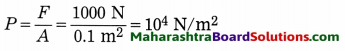The pressure = 10 N/m2.

Problem 4.
A metal block has thmensions 10 cm × 5 cm × 2 cm and the density of the metal is 8 × 103 kg/m3. It is kept on a table with the face 10 cm × 5 cm in contact with the table. Find the force and pressure exerted by the block on the table. (g = 9.8 m/s2)
Solution :
Data : 1 = 10 cm, b = 5 cm,
h = 2 cm, p= 8 × 10 kg/m3, g = 9.8 m/s2,
A = lb = 10cm × 5cm = 50cm2 = 50 × 104m2
= 5 × 10-5m2, force =?, pressure = ?
Volume of the block = lbh =
10 cm × 5 cm × 2 cm= 100 cm3
= 100 × 10-6 m3 = 1 × 10-4m3
Mass of the block = volume × density
(∵ density = mass/volume)
∴Mass of the block,
m = 1 × 10-4 m3× 8 × 103 kg/m3 = 0.8 kg
Weight of the block = mg = 0.8 kg × 9.8 m/s2 = 7.84 N
∴ The force exerted by the block on the table = 7.84 N.
force 7.84 N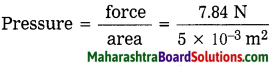= 1.568 × 103 N/m2 or 1.568 × 103 Pa
The pressure exerted by the block on the table = 1.568 × 10 N/m2 or 1.568 × 103 Pa.

Problem 5.
A body of volume loo cm3 is immersed completely in water. Find the weight of the water displaced by the body. 1g = 9.8 m/s2. p (water) = kg/m3]
Solution :
Data: V = 100 cm3 = 100 × 10-6 m3
= 1 × 10 m3, p(water) = 10 kg/m3
g = 9.8 m/s2, weight of the displaced water ?
Density = $$\frac{\text { mass }}{\text { volume }}$$
∴ Mass = volume × density
Volume of the water displaced by the body = l × 10-4 m3
∴Mass of the water displaced,
m = l × 10-4 m3 × 10 kg/m3 = 0.1 kg
∴Weight of the water displaced
= mg = 0.1 kg × 9.8 m/s2 = 0.98 N.
The weight of the water displaced by the body = 0.98 N.Problem 6.
A body of mass 200 g and volume 50 cm3 is put in a bucket containing water. Will it float or sink ?
[ρ(water) = 1 g/cm3]
Solution:
Data: m = 200 g, V= 50 cm3,
ρ (water) = 1 g/cm3
Density(ρ) = $$\frac{\text { mass }}{\text { volume }}$$
∴ ρ (body) = $$\frac{200}{50 \mathrm{~cm}^{3}}$$
It is greater than the density of water.
Hence, the body will sink in water.

Problem 7.
A body of mass 200 g and volume 400 cm3 is put in a bucket containing water. Will it float or sink?
[ρ (water)=1 g/cm3]
Solution:
Proceed as above.
ρ (body) = $$\frac{200 \mathrm{~g}}{400 \mathrm{~cm}^{3}}$$ = 0.5 g/cm3
It is less than the density of water.
∴ The body will float in water.

Problem 8.
The mass of a tile is 500 g. If the density of the tile is 2.5 g/cm3, what will be the weight of the tile when it is completely immersed in water?
(g = 9.8 m/s2, ρ(water) = 1000 kg/m3)
Solut10n:
Data: m = 500, g = 0.5 kg, ρ
(tile) = 2.5 g/cm3 = 2500 kg/m3, g = 9.8 m/s2,
ρ (water) = 1000 kg/m3, weight of the tile when completely immersed in water (also called the apparent weight) = ?
ρ = $$\frac{m}{V}$$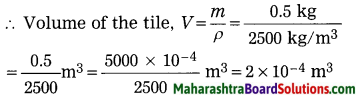∴ Volume of water displaced by the tile
= 2 × 10-4 m3
∴ Mass of water displaced by the tile
m’ = ρ (water) V = 1000 kg/m3 × 2 × 10-4 m3
= 0.2kg
∴ Magnitude of the weight of this water
= mg = 0.2 kg × 9.8 m/s2 = 1.96 N
∴ Buoyant force exerted on the tile = 1.96 N
Magnitude of the weight of the tile =
mg = 0.5 kg × 9.8 m/s2 = 4.9 N
∴ Weight of the tile when completely immersed in water (apparent weight) = weight of the tile in air-buoyant force on the tile
= 4.9 N – 1.96 N = 2.94 N(downward)Examples For Practice:

[g = 9.8 m/s2, ρ (water) = 103 kg/m3 = 1 g/cm3]

Question 1.
Calculate the relative density of a metal having density 7.5 g/cm3.
7.5

Question 2.
Find the density of steel if its relative density is 8 and the density of water is 10 kg/m3.
8 × 103 g/cm3

Question 3.
A body has mass 200 g and volume 100 cm3. Find its density and relative density.
2 g/cm3, 2

Question 4.
If the relative density of a material is 2.5, find its density.
2.5 × kg/m3 or 2.5 g/cm3

Question 5.
A force of 100 N is applied on an area 40 cm × 25cm. Find the corresponding pressure.
103 N/m2Question 6.
If the pressure exerted on an area 10 cm × 10 cm is 1000 dynes/cm2, find the applied force.
105 dynes

Question 7.
A metal block of mass 10 kg is kept on a table. If the contact surface area Is 100 cm2, find the pressure on the table.
9.8 × 103 N/m2 or 9.8 × 103 Pa

Question 8.
A body of volu.me 50 cm3 is immersed completely in water. Find the weight of the water displaced by the body.
0.49 N

Question 9.
A block of mass 100 g and volume 20 cm3 is put in a bucket filled with water. Will it float or sink?